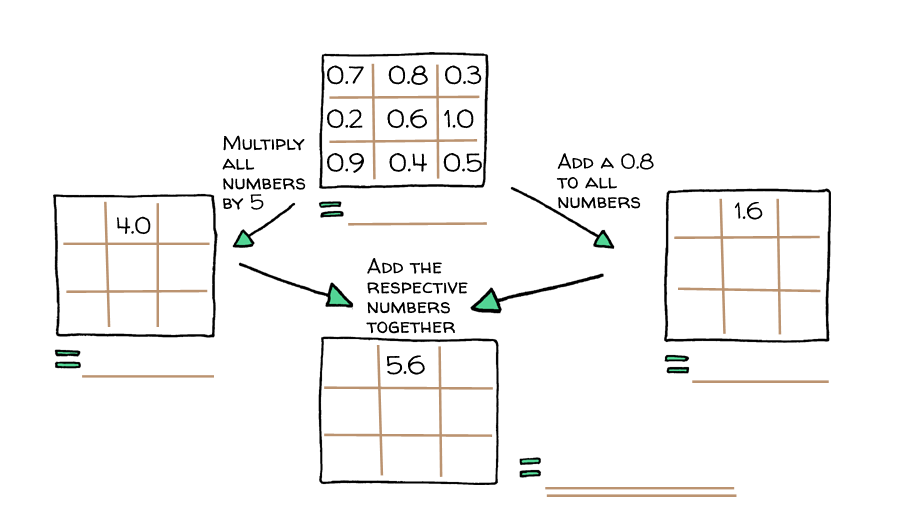# Magic Squares and Algebra (Investigation)

Lesson

## Magic SquaresSo what is so magical about these squares? They're just a bunch of numbers in little squares.

Still unsure? Here's a hint: Take a look at the rows, columns and diagonals.

That's right! A magic square is a square grid, generally filled with integers, where the rows, columns and main diagonals sum to the same thing, often known as the magic constant.

#### Example

The magic constant for the $3\times3$3×3 grid above is 15.
Rows

• $8+1+6=15$8+1+6=15
• $3+5+7=15$3+5+7=15
• $4+9+2=15$4+9+2=15

Columns

• $8+3+4=15$8+3+4=15
• $1+5+9=15$1+5+9=15
• $6+7+2=15$6+7+2=15

Main Diagonals

• $8+5+2=15$8+5+2=15
• $6+5+4=15$6+5+4=15

Another cool thing about magic squares is that when you add two magic squares together, they also form another magic square, like such:##### Question
1. What happens when you subtract them?
2. What if you multiply them?

The grid entries don't even have to be integers! Look at the following examples below with mixed numbers and decimals!##### Question

Can you fill in the missing numbers for the magic square on the right?##### Question

Can you fill in the above magic squares and find their magic constants?

##### Question

There are also magic squares with algebraic terms!

What is the magic constant in the magic square below?##### Questions

Look at the following squares:1. What kind of substitution are they asking you to make? Make the substitutions.
2. Are these magic squares? If they are, what are their magic constants?

##### Challenge Magic Square

Can you fill out the following magic square?